Contents >> Engineering Mathematics >> Hydro Mechanical Drives and Transmissions >> Dynamic Analysis >> Modeling of blocking mode >> General case of blocking of arbitrary number of clutches

Dynamic analysis of mechanical transmissions - General case of blocking of clutches

The general case of blocking of arbitrary number of clutches

Let's consider algorithm of definition of the moment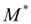values transmitted at blocking generally, i.e. at blocking any number of clutches, rigidly connected between them. In the beginning we’ll be limited to consideration of some simple examples.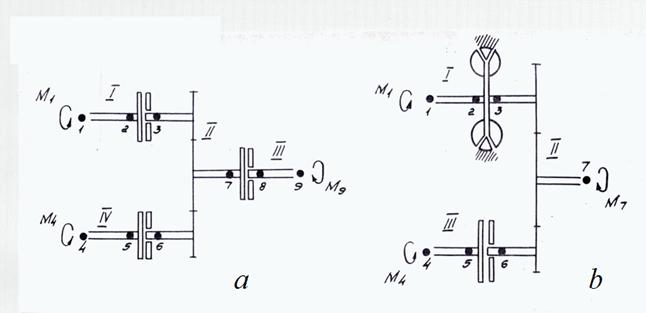Fig. 7. To a conclusion of the blocking equations

On Fig. 7 fragments of the drive schemes containing blocked elements rigidly connected with each other are presented:

1) parallel-serial connection of three frictional clutches (Fig. 7 а );

2) parallel connection of hydraulic torque converter and frictional clutch (Fig. 7 b ).

Let's consider first of all the scheme on Fig. 7 а . It consists of four sites. The dynamics equations of the scheme sites I – IV look like: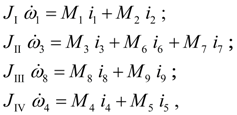(48)

here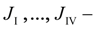the reduced moments of inertia of sites I ,..., IV ;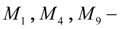the external (known) moments;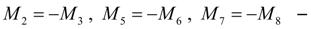the seeking moments transmitted by clutches; transfer ratios in nodes 1 – 8 are equal to: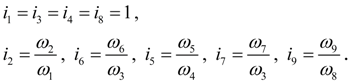(49)

Let in some time moment it has appeared: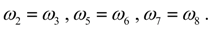Let’s agree that we’ll define seeking moments in nodes j of clutches, i.e.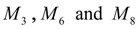.

Let's assume, that all three clutches are blocked, then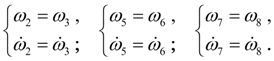(50)

Considering (49), from equations (48) and equality (50) we’ll receive the following system of the equations in unknowns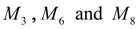: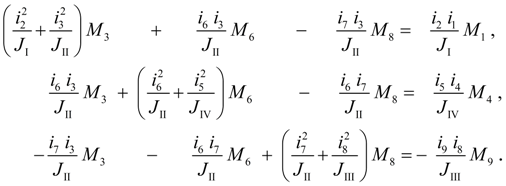(51)

Although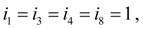they are written down in system of the equations (51) for a generality of algorithm.

Solving system of the equations (51), we’ll receive the seeking moments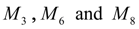. Then each of them we’ll substitute instead of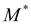in blocking conditions of friction clutch (15) and we’ll find out, what from these clutches is blocked. If for any frictional clutch the blocking conditions are not executed, then the blocking equations are formed and solved again, and the moment of that clutch for which the blocking conditions are not executed, passes in number of the external (known) moments, i.e. in the right part of the equations which order becomes, obviously, smaller. Process repeats until either all unknowns of system of the equations of a kind (51) will not satisfy to blocking conditions, or all considered clutches will be unblocked.

Now we’ll address to the scheme on Fig. 7 b . It consists of three sites. The dynamics equations of sites I – III look like: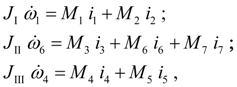(52)

where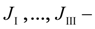the reduced moments of inertia of sites I ,..., III ;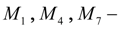the external (known) moments;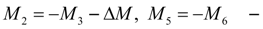the seeking moments transmitted by clutches;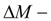the moment of losses on the hydraulic torque converter reactor blades in blocking mode [see explanatory comments to the equations (19)]; transfer ratios of nodes 1 – 7 are equal to: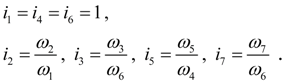(53)

Let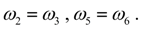As before, we’ll define the seeking moments in nodes j of clutches, i.e.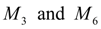. Let's assume, that both clutches are blocked, i.e.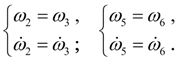(54)

Considering (53), from (52) and (54) we’ll receive the following blocking equations: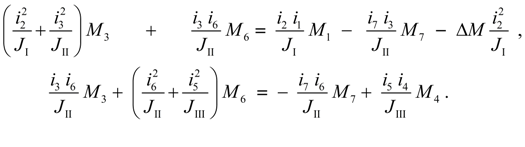(55)

Solving the equations (55) relatively, and substituting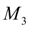in blocking conditions (19) for the hydraulic torque converter instead of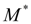, and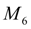in blocking conditions (15) for a friction clutch instead of, we’ll define, whether both clutches are blocked. If blocking conditions are executed, the found valuesare substituted in the corresponding dynamics equations of sites; if for any clutch these conditions are not executed, this clutch is excluded from consideration of blocking mode, its moment accepts other value defined from (19) or (15), and passes in number of the external moments. Process repeats until either there will be no system of clutches at which the moments received by the solution of the blocking equations, will satisfy to blocking conditions, or such clutches will not appear at all.

The considered examples allow to pass to formalization of algorithm of drawing up of blocking equations generally.

Let's preliminary note the following circumstances following from considered examples. Let some clutch at which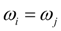, is considered and let the moments of inertia of the sites adjoining to given clutch in nodes i and j , are equal accordingly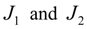, and transfer ratios in the same nodes are equal accordingly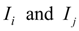then the coefficient at the unknown moment of given clutch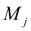in the equation received by equating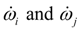will be equal to: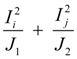.     (56)

Thus, all diagonal elements of a matrix of coefficients of the blocking equations have the form (56).

The unknown moments of others clutches, rigidly connected with the first, can also enter into the same equation. It means, that one of nodes of another clutch (let it will be node n ) enters into the site of the scheme containing either node i , or node j . Then the factor at the unknown moment of this clutch will be equal to: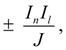(57)

where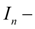transfer ratio in node n ;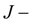moment of inertia of the site containing node n ;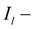transfer ratio: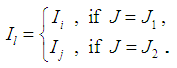(58)

The sign « + » undertakes, if either nodes j and n are included into one site and node n is initial, or units i and n are included into one site and unit n is final (i.e. these clutches are connected in parallel);  the sign « – » – otherwise (at consecutive connection of clutches).

If the moment in node n is known (it is possible in the two cases: either the unit n is not a node of clutch, or a clutch, containing unit n , is not blocked), then its value increased by coefficient of a kind (57), is transferred to the right part of the equation.

Formally process of drawing up of the blocking equations is reduced generally (at any number of the blocked elements rigidly connected with each other) to the following algorithm. First of all the all clutches which can be blocked, i.e. clutches at which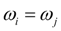, are defined and the additional information (tab. 2) is represented:

Tab 2. Information about clutches with possible blocking

 Numbers of clutches, which can be blocked Numbers of input ( i ) and output ( j ) nodes of a clutch: i j Numbers of boundary sites adjoining to the given clutch in nodes: i j 1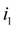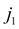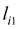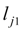2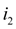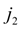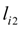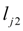3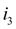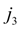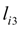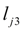. . . . . . . . . . . . . . . . . . . . . . . . . . . . . . . . . . . N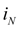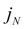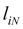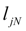A clutch with number т ( т = 1, ... , N ) is considered. Diagonal elements of a matrix of coefficients of the blocking equations are calculated from the formula: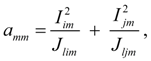(59)

where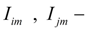transfer ratios in nodes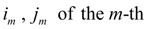clutch;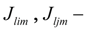moments of inertia of sites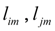, adjoining in nodes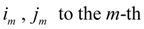clutch.

If the m -th clutch is rigidly connected with k -th clutch, then not diagonal element of a matrix of coefficients is equal to: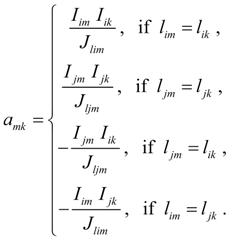(60)

The right parts of the blocking equations for m -th clutch are calculated in the form of: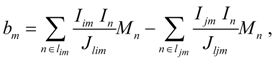(61)

where n – external nodes which are not entering into input ( i ) and output ( j ) nodes of clutches which can be blocked (tab. 2)

After detour of all N clutches the system of N linear blocking equations in N unknown moments is generated. Each moment received as a result of the solution of the blocking equations is necessary for checking up, whether it satisfies to blocking conditions of the given clutch. For this purpose it is necessary to substitute its value in corresponding given clutch blocking conditions (15), (19) or (20) instead of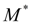. If the clutch is really blocked, it realizes the moment found from the blocking equations.

If the all moments satisfy to blocking conditions then process of drawing up and decision of the blocking equations comes to the end. If the moment of any clutch does not satisfy to blocking conditions, then this clutch is excluded from among blocked, and process of drawing up and the solution of the blocking equations repeats again. Thus the moment of not blocked clutch is equal to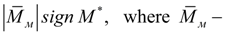the clutch moment, defined from its characteristic,– the moment of the same clutch, found from the blocking equations.

 < Previous Contents Next >